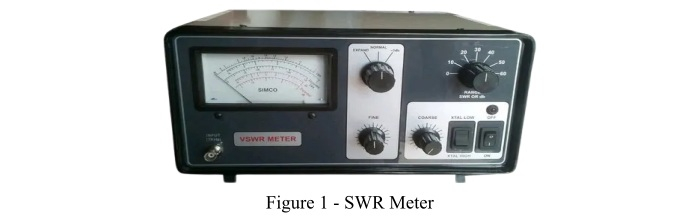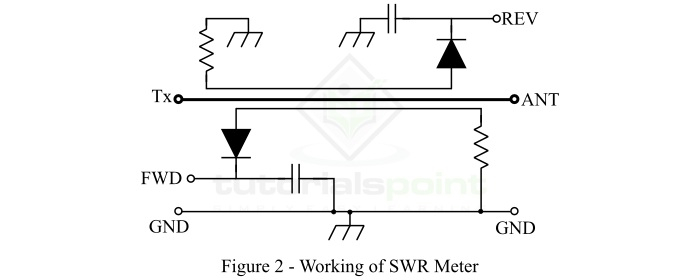# SWR Meter ŌĆō What is It & How to Use It

## What is SWR Meter?

SWR meter stands for Standing Wave Ratio meter, it is a measuring instrument used to measure the standing wave ratio (SWR) in a transmission line or transmitter feeder. It is also known as VSWR or ISWR. There are different types of SWR meters available, however, the working principle of all the SWR meters is the same. In this article, we will discuss the SWR meter along with its working and applications.The SWR meter measures the degree of mismatch between a transmitter feeder and its antenna (load). This measurement is used to know the effectiveness of the impedance matching in the system.

SWR meter provides information about the amount of radio frequency (RF) energy that is reflected back to the transmitter as compared to the amount of radio frequency energy that the transmitter sent during the operation. This ratio should not be high. The ideal ratio is 1:1, which indicates that the energy is reached the destination and no energy is reflected back to the transmitter.

In the radio industry, the most common type of SWR meter used contains a dual-directional coupler. It samples a small amount of energy in one direction and a diode is provided in the circuit to rectify it before applying it to the SWR meter. However, when we use an SWR meter with a single directional coupler, then the coupler is to be rotated at 180┬░ for sampling the power which is coming out from two different directions. The forward and reflected powers sampled by the single coupler are then used to determine the standing wave ratio. Sometimes, two single-directional couplers are provided one for each direction.

The SWR meter is used at very high frequencies, but cannot be used at low frequencies due to impractical long transmission lines.

## What is the Standing Wave Ratio?

Before discussing how to use a SWR meter, it is fruitful to get the basic knowledge about standing wave ratio (SWR). The SWR may be defined as under ŌłÆ

• The standing wave ratio (SWR) is defined as the ratio of the highest radio-frequency voltage to the minimum radio-frequency voltage through the transmission line. Here, the standing wave ratio is determined in terms of the voltage of the transmission line. Therefore, it is called the voltage standing wave ratio (VSWR).

• The standing wave ratio (SWR) may also be defined as the ratio of the highest ratio-frequency current to the lowest radio-frequency current through the transmission line. Since, in this case, the SWR is identified in terms of line current, hence it is called current standing wave ratio (ISWR).

## Working of SWR Meter

To understand the working of the SWR meter, we consider a directional SWR meter as shown in Figure2. The directional SWR meter is used to identify the amplitude of the transmitted wave and the amplitude of the reflected wave.This SWR meter consists of a transmitter (Tx) terminal and an antenna (ANT) terminal which are connected through a transmission line. Here, the directional couplers are used to couple the transmission line electromagnetically. Resistors are used to terminate the transmission line at one end whereas diodes are used at another end of the line for modification. Here, resistors help in matching the characteristic impedance of the transmission line, whereas diodes assist in changing the amplitude of the signal to their corresponding DC voltage, which is then smoothed by the filter capacitors.

## How to Use SWR Meter?

Using an SWR meter is quite simple; the following steps are to be followed ŌłÆ

• Step 1 ŌłÆ Find a clear channel or frequency.

• Step 2 ŌłÆ Reduce the output power from the transmitter.

• Step 3 ŌłÆ Set the mode switch to a mode like CW, AM, FM, etc. where a constant output is produced.

• Step 4 ŌłÆ Set the VSWR meter switch provided on the front panel to FORWARD, and rotate the CAL knob down to prevent the overloading of the meter.

• Step 5 ŌłÆ When the transmitter is transmitting, adjust the CAL knob to obtain a full-scale reading.

• Step 6 ŌłÆ With the SWR meter calibrated for the forward power, switch the meter to the reverse position and take the reading.

• Step 7 ŌłÆ Stop transmitting, and check on other frequencies in the same way.

## SWR Formula

The standing wave ratio (SWR) is calculated by the following expression ŌłÆ

$$\mathrm{SWR = \frac{1+\left|\Gamma \right|}{1-\left|\Gamma \right|}}$$

Where, ╬ō is called the reflection coefficient, and is given by

$$\mathrm{\Gamma = \frac{V_{rev}}{V_{fwd}}}$$

## Applications of SWR Meter

The following are some important applications of the SWR meter ŌłÆ

• SWR meter is used for the installation and tuning of transmitting antennas.

• SWR meter is also used in microwave-based medical applications like microwaveelectro surgery.

• In electronics, the SWR meter is used for adjusting radio transmitter and their antennas and feedlines.

## Conclusion

In this article, we discussed the SWR meter, its working, formula and applications. A major limitation of the SWR meter is that it does not measure the actual impedance of the load, but only the mismatch ratio. Therefore, to measure the actual impedance, we need a radio frequency measuring device like an antenna analyzer.